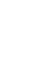## 4.1 Introduction

The first discovery of a high-superconductor in 1986  initiated a surge of interest in new materials which have a higher superconducting transition temperature (). As a result of vital experimental researches, various copper oxides, doped with charges, have been identified as a high-superconductor. One remarkable structural similarity among these high-cuprates is the existence of the CuO2 planes, and there have been many theoretical studies trying to explain the superconductivity as a nature of the doped CuO2 square lattice. Still, the problem of correlated electrons on the two-dimensional lattice is difficult, and no rigorous solution to the problem has been found.

For the one-dimensional chain system, on the other hand, quite a few things are known exactly. For example, the antiferromagnetic Heisenberg model of the S=1/2 spin-chain was exactly solved by H. A. Bethe in 1931 , and using a similar technique, the one dimensional Hubbard model, as well as themodel in special cases (J=0 and 2t) have also been solved [42,43]. Considering these successes in one-dimensional systems, one approach to tackle the superconducting cuprates is to investigate the quasi one-dimensional lattices known as `spin ladder' structures (Fig.25a), which are strips of square lattice with a finite width and infinite length.Early theoretical works of the spin ladder system were numerical studies on isolated 2-leg spin ladders. For the Heisenberg model of a 2-leg spin ladder, a many-body singlet ground state was suggested, with a finite energy gap (spin-gap) to the excited states [20,44]. The ground state structure is mainly composed of spin singlet pairs on the rungs, as shown in Fig.25b . The hole doped 2-leg ladders have also been investigated theoretically, in relation to the superconductivity [45,46,47]. These works suggest that two doped holes will form a bound state so that the surrounding spins can remain spin singlet. The paired holes, which behave as hard-core bosons, were suggested to take a superconducting ground state [45,46].

Behavior of wider spin-ladder systems was first emphasized by T. M. Rice et al. in 1993 . In Ref. , the authors first pointed out that the homologous series of cuprate Srn-1Cun+1O2n should realize the N-leg ladder structure, and pointed out contrasting magnetic behavior between the even-number-leg ladders and the odd-number-leg ladders. The even-number-leg ladders were to manifest a spin-gap, while the odd-number-leg system should be gapless. This idea of alternating ground states has been supported by numerical simulations of the spin ladders up to 4-legs  and a mean-field calculation of the 2-leg and the 4-leg ladder systems .